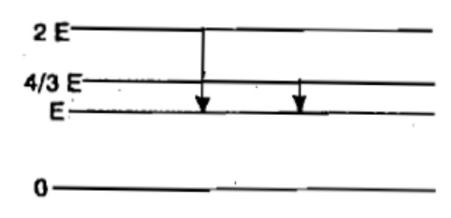The ground state energy of H-atom is 13.6 eV. The energy needed to ionise H-atom from its second excited state 

(1) 1.51 eV          (2) 3.4 eV            (3) 13.6 eV             (4) 12.1 eV

Concept Questions :-

Bohr's model of atom
High Yielding Test Series + Question Bank - NEET 2020

Difficulty Level:

The element which has a ${k}_{a}$ x-rays line of wavelength 1.8 $\stackrel{0}{A}$ is

(1) Co, Z=27      (2) Iron, Z = 26        (3) Mn, Z = 25           (4) Ni, Z = 28

Concept Questions :-

Spectral series
High Yielding Test Series + Question Bank - NEET 2020

Difficulty Level:

The given diagram indicates the energy levels of a certain atom. When the system moves from 2E level to E, a photon of wavelength $\lambda$ is emitted. The wavelength of photon produced during its transition from $\frac{4E}{3}$ level to E is(1) $\frac{\lambda }{3}$           (2) $\frac{3\lambda }{4}$        (3) $\frac{4\lambda }{3}$            (4) $3\lambda$

Concept Questions :-

Spectral series
High Yielding Test Series + Question Bank - NEET 2020

Difficulty Level:

The wavelength of the first spectral line in the Balmer series of hydrogen atom is 6561 $\stackrel{0}{A}$. The wavelength of the second spectral line in the Balmer series of singly ionized helium atom is [IIT-JEE 2011]

(1) 1215 $\stackrel{0}{A}$        (2) 1640 $\stackrel{0}{A}$            (3) 2430 $\stackrel{0}{A}$           (4) 4687 $\stackrel{0}{A}$

Concept Questions :-

Spectral series
High Yielding Test Series + Question Bank - NEET 2020

Difficulty Level:

Energy E of a hydrogen atom with principal quantum number n is given by $E=\frac{-13.6}{{n}^{2}}eV$.  The energy of a photon ejected when the electron jumps from n = 3 state to n = 2 state of hydrogen, is approximately 

(1) 1.5 eV         (2) 0.85 eV          (3) 3.4 eV            (4) 1.9 eV

Concept Questions :-

Bohr's model of atom
High Yielding Test Series + Question Bank - NEET 2020

Difficulty Level:

The energy of ground electronic state of hydrogen atom is -13.6 eV.  The energy of the first excited state will be 

(1) -54.4 eV         (2) -27.2 eV           (3) -6.8 eV               (4) -3.4 eV

Concept Questions :-

Bohr's model of atom
High Yielding Test Series + Question Bank - NEET 2020

Difficulty Level:

The total energy of an electron in the first excited state of hydrogen is about -3.4 eV. Its kinetic energy in this state is 

(1) -3.4 eV            (2) -6.8 eV             (3) 6.8 eV           (4) 3.4 eV

Concept Questions :-

Bohr's model of atom
High Yielding Test Series + Question Bank - NEET 2020

Difficulty Level:

The energy of an electron in excited hydrogen atom is -3.4 eV. Then according to Bohr's theory, the angular momentum of the electron in Js is [Kerala PET 2002]

(1)

(2)

(3)

(4)

(5)

Concept Questions :-

Bohr's model of atom
High Yielding Test Series + Question Bank - NEET 2020

Difficulty Level:

When hydrogen atom is in its first excited level, its radius is 

(1) four times, its ground state radius

(2) twice, its ground state radius

(3) same as its ground state radius

(4) half of its ground state radius

Concept Questions :-

Bohr's model of atom
High Yielding Test Series + Question Bank - NEET 2020

Difficulty Level:

The ionisation potential of helium atom is 24.6 volt, the energy required to ionise it will be

(1) 24.6 eV            (2) 24.6 volt          (3) 13.6 volt                 (4) 13..6 eV

Concept Questions :-

Various atomic model